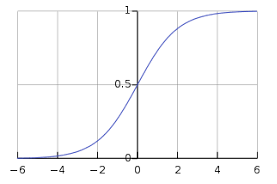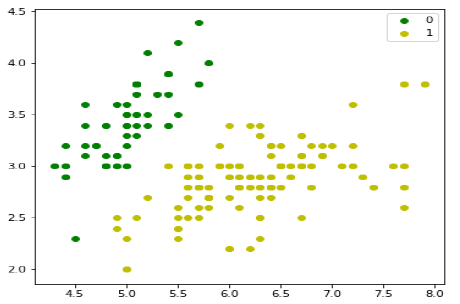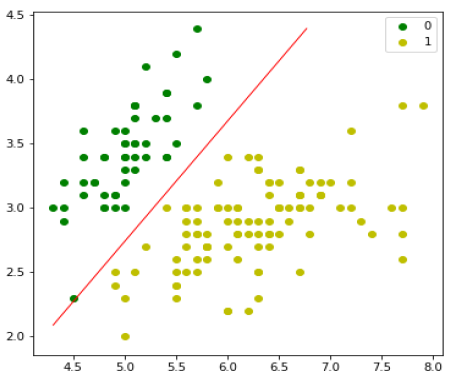# Binary Logistic Regression Model of ML

The simplest form of logistic regression is binary or binomial logistic regression in which the target or dependent variable can have only 2 possible types either 1 or 0. It allows us to model a relationship between multiple predictor variables and a binary/binomial target variable. In case of logistic regression, the linear function is basically used as an input to another function such as 𝑔 in the following relation −

$$h_{\emptyset}(x) = g(\emptyset^{T}x)where 0\leq h_{\emptyset}\leq1$$

Here, 𝑔 is the logistic or sigmoid function which can be given as follows −

$$g(z) = \frac{1}{1+e^{-z}} where\: z=\:\emptyset^{T}x$$

To sigmoid curve can be represented with the help of following graph. We can see the values of y-axis lie between 0 and 1 and crosses the axis at 0.5.The classes can be divided into positive or negative. The output comes under the probability of positive class if it lies between 0 and 1. For our implementation, we are interpreting the output of hypothesis function as positive if it is ≥0.5, otherwise negative.

We also need to define a loss function to measure how well the algorithm performs using the weights on functions, represented by theta as follows −

$$h\:=\:g(X\emptyset)$$

$$J(\emptyset)\:=\:\frac{1}{m}.(-y^{T}log(h)\:-\:(1-y)^{T}\:log(1-h)$$

Now, after defining the loss function our prime goal is to minimize the loss function. It can be done with the help of fitting the weights which means by increasing or decreasing the weights. With the help of derivatives of the loss function w.r.t each weight, we would be able to know what parameters should have high weight and what should have smaller weight.

The following gradient descent equation tells us how loss would change if we modified the parameters −

$$\frac{\partial J(\emptyset)}{\partial \emptyset_{j}}\:=\:\frac{1}{m}\:X^{T}\:(g(X\emptyset)\:-y)$$

## Implementation in Python

Now we will implement the above concept of binomial logistic regression in Python. For this purpose, we are using a multivariate flower dataset named ‘iris’ which have 3 classes of 50 instances each, but we will be using the first two feature columns. Every class represents a type of iris flower.

First, we need to import the necessary libraries as follows −

import numpy as np
import matplotlib.pyplot as plt
import seaborn as sns
from sklearn import datasets


Next, load the iris dataset as follows −

iris = datasets.load_iris()
X = iris.data[:, :2]
y = (iris.target != 0) * 1


We can plot our training data as follows −

plt.figure(figsize=(6, 6))
plt.scatter(X[y == 0][:, 0], X[y == 0][:, 1], color='g', label='0')
plt.scatter(X[y == 1][:, 0], X[y == 1][:, 1], color='y', label='1')
plt.legend();Next, we will define sigmoid function, loss function and gradient descend as follows −

class LogisticRegression:
def __init__(self, lr = 0.01, num_iter = 100000, fit_intercept = True, verbose = False):
self.lr = lr
self.num_iter = num_iter
self.fit_intercept = fit_intercept
self.verbose = verbose
intercept = np.ones((X.shape, 1))
return np.concatenate((intercept, X), axis=1)
def __sigmoid(self, z):
return 1 / (1 + np.exp(-z))
def __loss(self, h, y):
return (-y * np.log(h) - (1 - y) * np.log(1 - h)).mean()
def fit(self, X, y):
if self.fit_intercept:


Now, initialize the weights as follows −

self.theta = np.zeros(X.shape)
for i in range(self.num_iter):
z = np.dot(X, self.theta)
h = self.__sigmoid(z)
gradient = np.dot(X.T, (h - y)) / y.size

z = np.dot(X, self.theta)
h = self.__sigmoid(z)
loss = self.__loss(h, y)

if(self.verbose ==True and i % 10000 == 0):
print(f'loss: {loss} \t')


With the help of the following script, we can predict the output probabilities −

def predict_prob(self, X):
if self.fit_intercept:
return self.__sigmoid(np.dot(X, self.theta))

def predict(self, X):
return self.predict_prob(X).round()


Next, we can evaluate the model and plot it as follows −

model = LogisticRegression(lr = 0.1, num_iter = 300000)
preds = model.predict(X)
(preds == y).mean()

plt.figure(figsize = (10, 6))
plt.scatter(X[y == 0][:, 0], X[y == 0][:, 1], color = 'g', label = '0')
plt.scatter(X[y == 1][:, 0], X[y == 1][:, 1], color = 'y', label = '1')
plt.legend()

x1_min, x1_max = X[:,0].min(), X[:,0].max(),
x2_min, x2_max = X[:,1].min(), X[:,1].max(),
xx1, xx2 = np.meshgrid(np.linspace(x1_min, x1_max), np.linspace(x2_min, x2_max))
grid = np.c_[xx1.ravel(), xx2.ravel()]
probs = model.predict_prob(grid).reshape(xx1.shape)
plt.contour(xx1, xx2, probs, [0.5], linewidths=1, colors='red');machine_learning_with_python_classification_algorithms_logistic_regression.htm